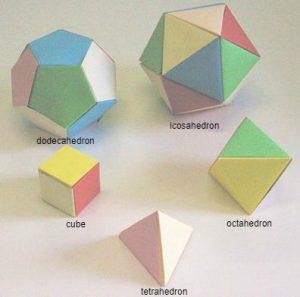A regular polygon has all its angles equal and all edge lengths equal. In a regular polyhedron all the faces are congruent regular polygons and the same number of polygons meet at each vertex.

Each regular polyhedron has its own codename. The tetrahedron is 333, the octahedron is 3333, the icosahedron is 33333, the cube is 444, and the dodecahedron is 555.

1. Can you crack the code and explain why these codenames have been given to the polyhedra?
2. Use scrap paper, follow these instructions to make the 5 regular polyhedra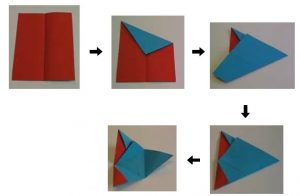TETRAHEDRON Fold an A4 sheet of paper as shown to make a tetrahedron net. Make another identical net, then slot the two nets together to form a rigid tetrahedron.

[Note: Use scrap paper. The standard size A4 office paper, used very widely worldwide (but not in the USA) has edge lengths in the ratio √2 to 1. Other sizes are in the same proportion, bigger sizes A1, A2 and A3, and smaller sizes A5, A6 etc.  If you do not have A4 paper you can cut your paper in this proportion to make the models.]

DODECAHEDRON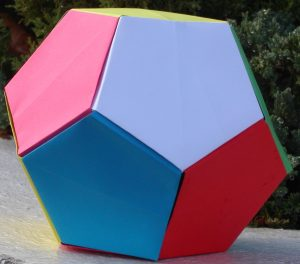Fold along the red line so that A touches O. Fold C to O similarly.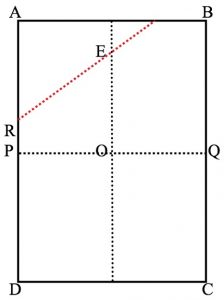Fold B and D to O. Next fold along PQ. Tuck the flap from corner D behind the flat from corner B to make ‘pockets’. Fold R and S up to the centre line EO to make pentagon.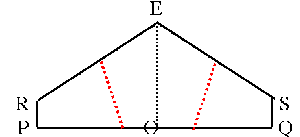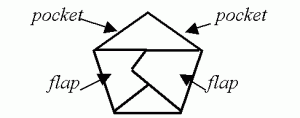Make 12 pentagons in this way. Assemble them to make a dodecahedron tucking all the flaps into the pockets of adjoining faces.

ICOSAHEDRON From the final stage of the tetrahedron recipe, make the folds shown below to produce a truncated tetrahedron. Make twenty of these truncated tetrahedra and glue them together to form an icosahedron.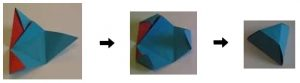Click here for the POLYHEDRA THROUGH PAPER FOLDING Inclusion and Home Learning Guide